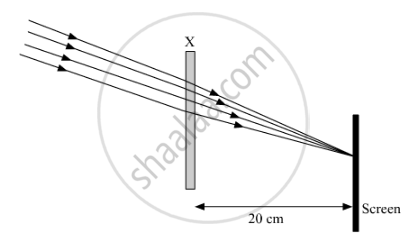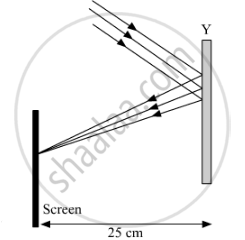Share

# Study the given ray diagrams and select the correct statement from the following - CBSE Class 10 - Science

ConceptRefraction of Light Image Formation by Lenses - Convex Lens

#### Question

Study the given ray diagrams and select the correct statement from the following:(A) Device X is a concave mirror and device Y is a convex lens, whose focal lengths are 20 cm and 25 cm respectively.
(B) Device X is a convex lens and device Y is a concave mirror, whose focal lengths are 10 cm and 25 cm respectively.
(C) Device X is a concave lens and device Y is a convex mirror, whose focal lengths are 20 cm and 25 cm respectively.
(D) Device X is a convex lens and device Y is a concave mirror, whose focal lengths are 20 cm and 25 cm respectively.

#### Solution

Device X is convex lens. This is because for a convex lens light rays which are parallel to each other but not to the principle axis meet on the focal plane. Also, the distance between the focal plane and the lens gives the focal length of the lens. Thus the focal length of the device X is 20 cm.

Device Y is concave mirror. ​This is because for a concave mirror light rays which are parallel to each other but not to the principle axis meet on the focal plane. Also, the distance between the focal plane and the lens gives the focal length of the mirror. Thus the focal length of the device Y is 25 cm.

Hence, the correct answer is Device X is a convex lens and device Y is a concave mirror, whose focal lengths are 20 cm and 25 cm respectively.

Is there an error in this question or solution?

#### Video TutorialsVIEW ALL 

Solution Study the given ray diagrams and select the correct statement from the following Concept: Refraction of Light - Image Formation by Lenses - Convex Lens.
S